W3cubDocs

/scikit-learn

class sklearn.ensemble.GradientBoostingClassifier(loss=’deviance’, learning_rate=0.1, n_estimators=100, subsample=1.0, criterion=’friedman_mse’, min_samples_split=2, min_samples_leaf=1, min_weight_fraction_leaf=0.0, max_depth=3, min_impurity_decrease=0.0, min_impurity_split=None, init=None, random_state=None, max_features=None, verbose=0, max_leaf_nodes=None, warm_start=False, presort=’auto’, validation_fraction=0.1, n_iter_no_change=None, tol=0.0001) [source]

Gradient Boosting for classification.

GB builds an additive model in a forward stage-wise fashion; it allows for the optimization of arbitrary differentiable loss functions. In each stage n_classes_ regression trees are fit on the negative gradient of the binomial or multinomial deviance loss function. Binary classification is a special case where only a single regression tree is induced.

Read more in the User Guide.

Notes

The features are always randomly permuted at each split. Therefore, the best found split may vary, even with the same training data and max_features=n_features, if the improvement of the criterion is identical for several splits enumerated during the search of the best split. To obtain a deterministic behaviour during fitting, random_state has to be fixed.

References

J. Friedman, Greedy Function Approximation: A Gradient Boosting Machine, The Annals of Statistics, Vol. 29, No. 5, 2001.

1. Friedman, Stochastic Gradient Boosting, 1999

T. Hastie, R. Tibshirani and J. Friedman. Elements of Statistical Learning Ed. 2, Springer, 2009.

Methods

 apply(X) Apply trees in the ensemble to X, return leaf indices. decision_function(X) Compute the decision function of X. fit(X, y[, sample_weight, monitor]) Fit the gradient boosting model. get_params([deep]) Get parameters for this estimator. predict(X) Predict class for X. predict_log_proba(X) Predict class log-probabilities for X. predict_proba(X) Predict class probabilities for X. score(X, y[, sample_weight]) Returns the mean accuracy on the given test data and labels. set_params(**params) Set the parameters of this estimator. staged_decision_function(X) Compute decision function of X for each iteration. staged_predict(X) Predict class at each stage for X. staged_predict_proba(X) Predict class probabilities at each stage for X.
__init__(loss=’deviance’, learning_rate=0.1, n_estimators=100, subsample=1.0, criterion=’friedman_mse’, min_samples_split=2, min_samples_leaf=1, min_weight_fraction_leaf=0.0, max_depth=3, min_impurity_decrease=0.0, min_impurity_split=None, init=None, random_state=None, max_features=None, verbose=0, max_leaf_nodes=None, warm_start=False, presort=’auto’, validation_fraction=0.1, n_iter_no_change=None, tol=0.0001) [source]
apply(X) [source]

Apply trees in the ensemble to X, return leaf indices.

New in version 0.17.

Parameters: X : {array-like, sparse matrix}, shape (n_samples, n_features) The input samples. Internally, its dtype will be converted to dtype=np.float32. If a sparse matrix is provided, it will be converted to a sparse csr_matrix. X_leaves : array-like, shape (n_samples, n_estimators, n_classes) For each datapoint x in X and for each tree in the ensemble, return the index of the leaf x ends up in each estimator. In the case of binary classification n_classes is 1.
decision_function(X) [source]

Compute the decision function of X.

Parameters: X : {array-like, sparse matrix}, shape (n_samples, n_features) The input samples. Internally, it will be converted to dtype=np.float32 and if a sparse matrix is provided to a sparse csr_matrix. score : array, shape (n_samples, n_classes) or (n_samples,) The decision function of the input samples. The order of the classes corresponds to that in the attribute classes_. Regression and binary classification produce an array of shape [n_samples].
feature_importances_
Return the feature importances (the higher, the more important the
feature).
Returns: feature_importances_ : array, shape (n_features,)
fit(X, y, sample_weight=None, monitor=None) [source]

Fit the gradient boosting model.

Parameters: X : {array-like, sparse matrix}, shape (n_samples, n_features) The input samples. Internally, it will be converted to dtype=np.float32 and if a sparse matrix is provided to a sparse csr_matrix. y : array-like, shape (n_samples,) Target values (strings or integers in classification, real numbers in regression) For classification, labels must correspond to classes. sample_weight : array-like, shape (n_samples,) or None Sample weights. If None, then samples are equally weighted. Splits that would create child nodes with net zero or negative weight are ignored while searching for a split in each node. In the case of classification, splits are also ignored if they would result in any single class carrying a negative weight in either child node. monitor : callable, optional The monitor is called after each iteration with the current iteration, a reference to the estimator and the local variables of _fit_stages as keyword arguments callable(i, self, locals()). If the callable returns True the fitting procedure is stopped. The monitor can be used for various things such as computing held-out estimates, early stopping, model introspect, and snapshoting. self : object
get_params(deep=True) [source]

Get parameters for this estimator.

Parameters: deep : boolean, optional If True, will return the parameters for this estimator and contained subobjects that are estimators. params : mapping of string to any Parameter names mapped to their values.
n_features

DEPRECATED: Attribute n_features was deprecated in version 0.19 and will be removed in 0.21.

predict(X) [source]

Predict class for X.

Parameters: X : {array-like, sparse matrix}, shape (n_samples, n_features) The input samples. Internally, it will be converted to dtype=np.float32 and if a sparse matrix is provided to a sparse csr_matrix. y : array, shape (n_samples,) The predicted values.
predict_log_proba(X) [source]

Predict class log-probabilities for X.

Parameters: X : {array-like, sparse matrix}, shape (n_samples, n_features) The input samples. Internally, it will be converted to dtype=np.float32 and if a sparse matrix is provided to a sparse csr_matrix. p : array, shape (n_samples, n_classes) The class log-probabilities of the input samples. The order of the classes corresponds to that in the attribute classes_. AttributeError If the loss does not support probabilities.
predict_proba(X) [source]

Predict class probabilities for X.

Parameters: X : {array-like, sparse matrix}, shape (n_samples, n_features) The input samples. Internally, it will be converted to dtype=np.float32 and if a sparse matrix is provided to a sparse csr_matrix. p : array, shape (n_samples, n_classes) The class probabilities of the input samples. The order of the classes corresponds to that in the attribute classes_. AttributeError If the loss does not support probabilities.
score(X, y, sample_weight=None) [source]

Returns the mean accuracy on the given test data and labels.

In multi-label classification, this is the subset accuracy which is a harsh metric since you require for each sample that each label set be correctly predicted.

Parameters: X : array-like, shape = (n_samples, n_features) Test samples. y : array-like, shape = (n_samples) or (n_samples, n_outputs) True labels for X. sample_weight : array-like, shape = [n_samples], optional Sample weights. score : float Mean accuracy of self.predict(X) wrt. y.
set_params(**params) [source]

Set the parameters of this estimator.

The method works on simple estimators as well as on nested objects (such as pipelines). The latter have parameters of the form <component>__<parameter> so that it’s possible to update each component of a nested object.

Returns: self
staged_decision_function(X) [source]

Compute decision function of X for each iteration.

This method allows monitoring (i.e. determine error on testing set) after each stage.

Parameters: X : {array-like, sparse matrix}, shape (n_samples, n_features) The input samples. Internally, it will be converted to dtype=np.float32 and if a sparse matrix is provided to a sparse csr_matrix. score : generator of array, shape (n_samples, k) The decision function of the input samples. The order of the classes corresponds to that in the attribute classes_. Regression and binary classification are special cases with k == 1, otherwise k==n_classes.
staged_predict(X) [source]

Predict class at each stage for X.

This method allows monitoring (i.e. determine error on testing set) after each stage.

Parameters: X : {array-like, sparse matrix}, shape (n_samples, n_features) The input samples. Internally, it will be converted to dtype=np.float32 and if a sparse matrix is provided to a sparse csr_matrix. y : generator of array of shape (n_samples,) The predicted value of the input samples.
staged_predict_proba(X) [source]

Predict class probabilities at each stage for X.

This method allows monitoring (i.e. determine error on testing set) after each stage.

Parameters: X : {array-like, sparse matrix}, shape (n_samples, n_features) The input samples. Internally, it will be converted to dtype=np.float32 and if a sparse matrix is provided to a sparse csr_matrix. y : generator of array of shape (n_samples,) The predicted value of the input samples.

3.2.4.3.5.1. Examples using sklearn.ensemble.GradientBoostingClassifier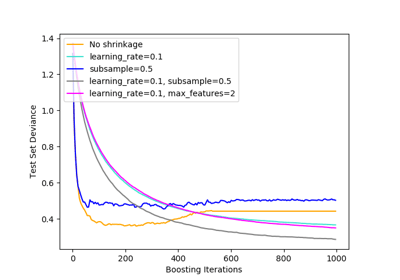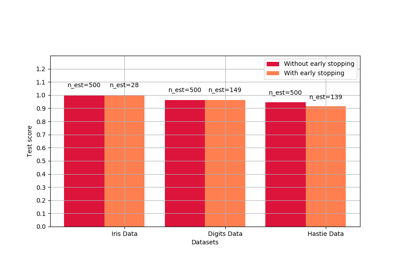Early stopping of Gradient Boosting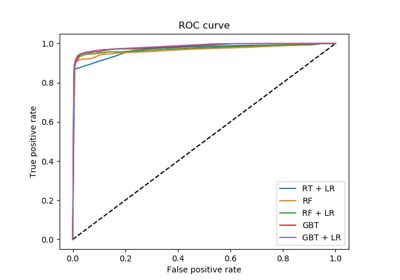Feature transformations with ensembles of trees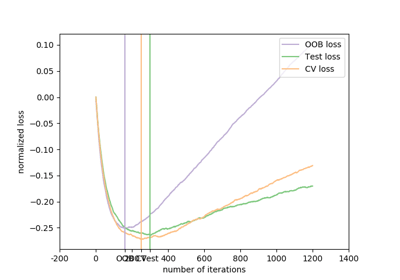Gradient Boosting Out-of-Bag estimates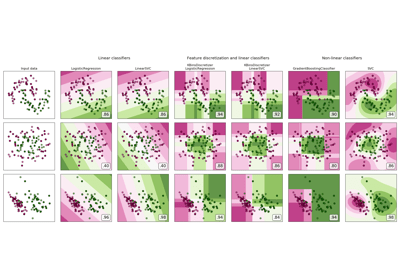Feature discretization

© 2007–2018 The scikit-learn developers